CBSE Class 10 Sample Paper for 2019 Boards

Class 10
Solutions of Sample Papers for Class 10 Boards

Question 30

If sec θ + tan θ = p, then find the value of cosec θ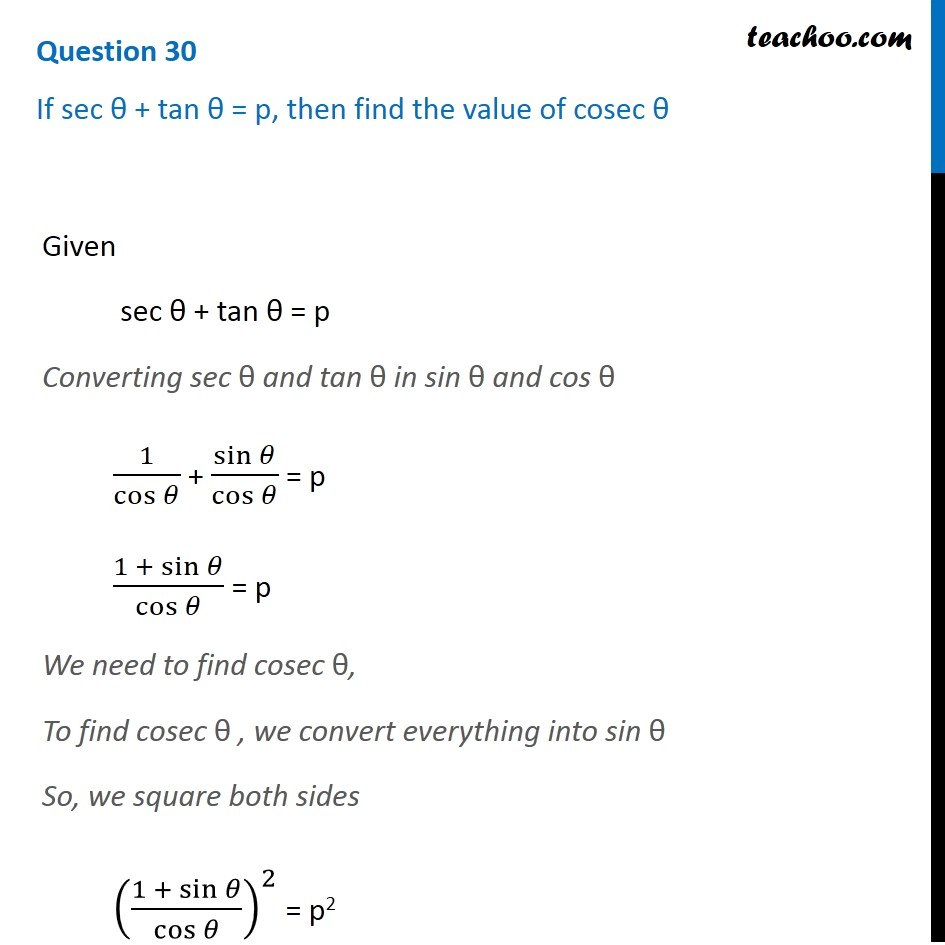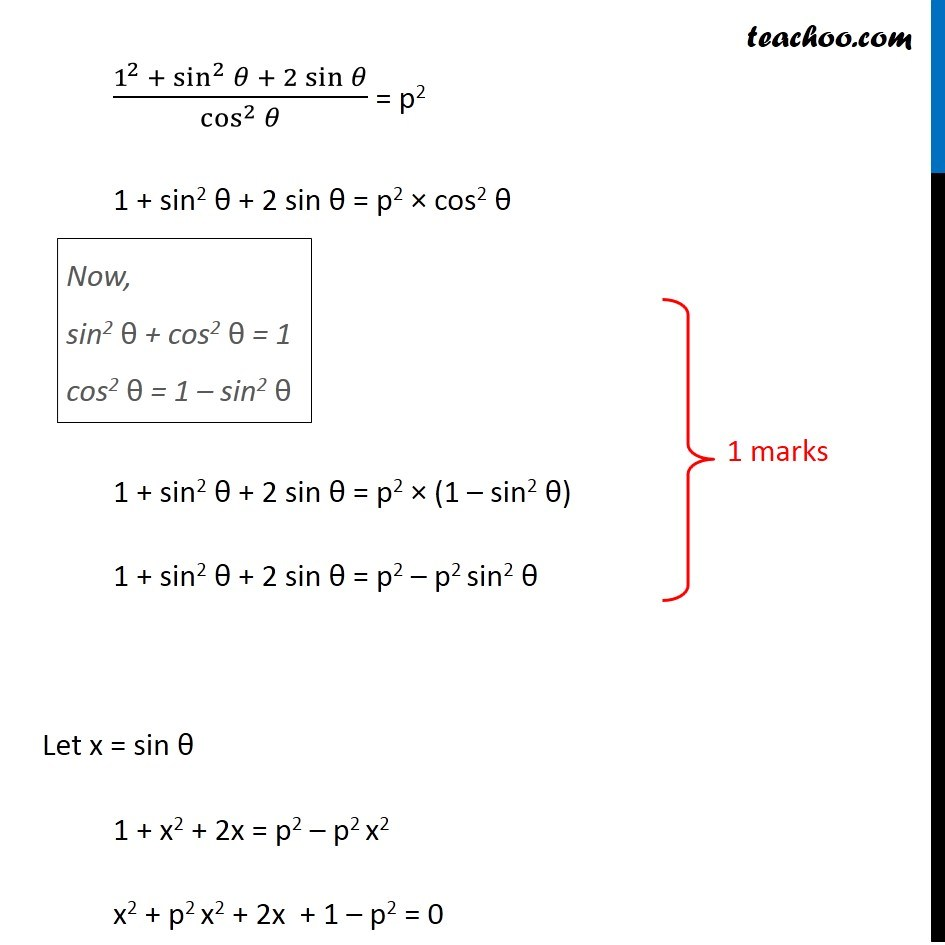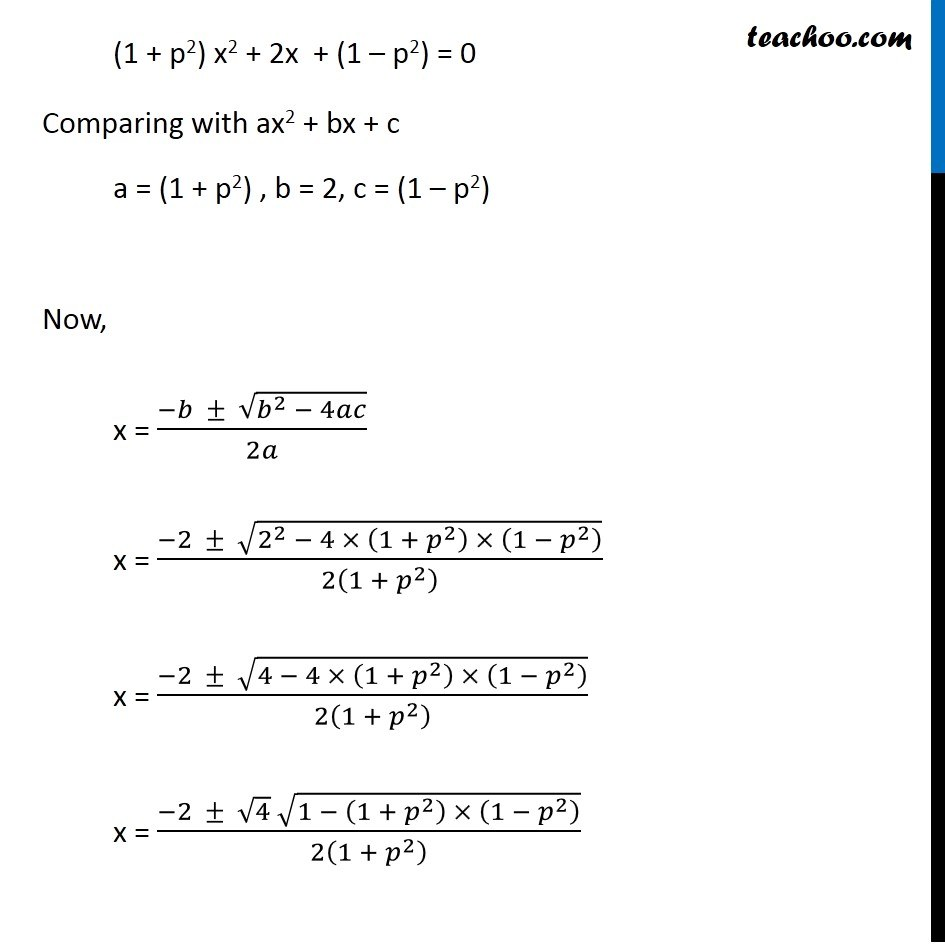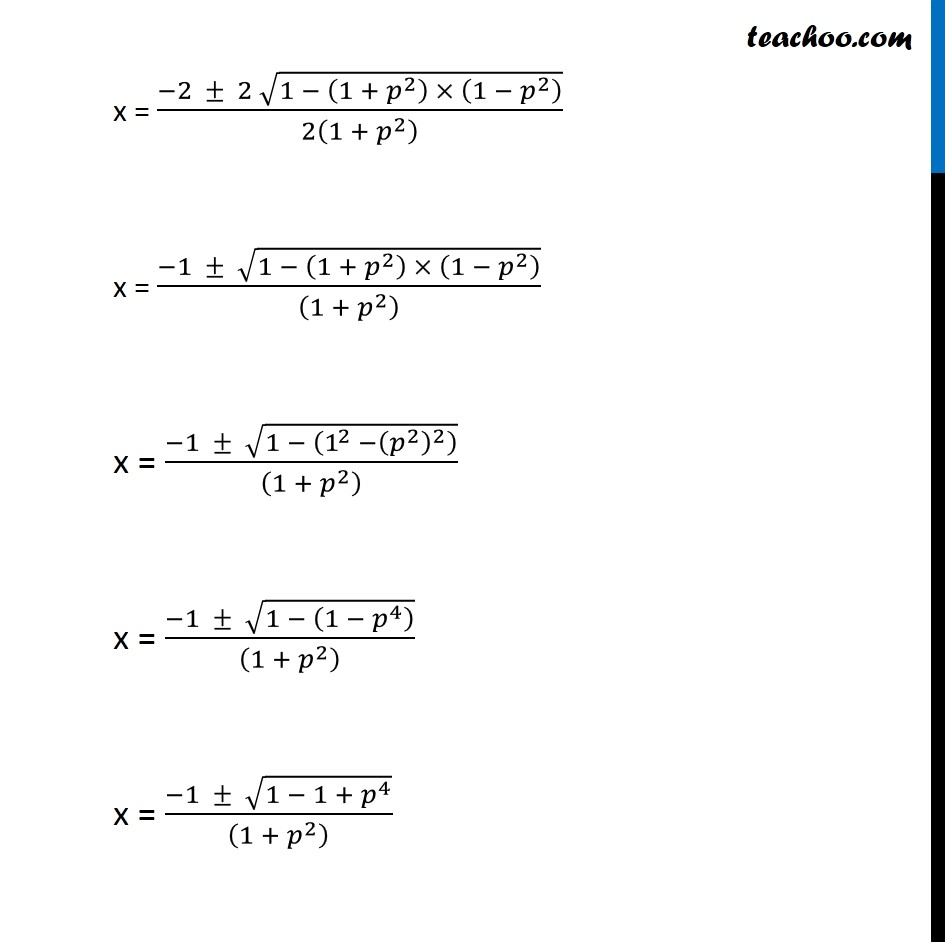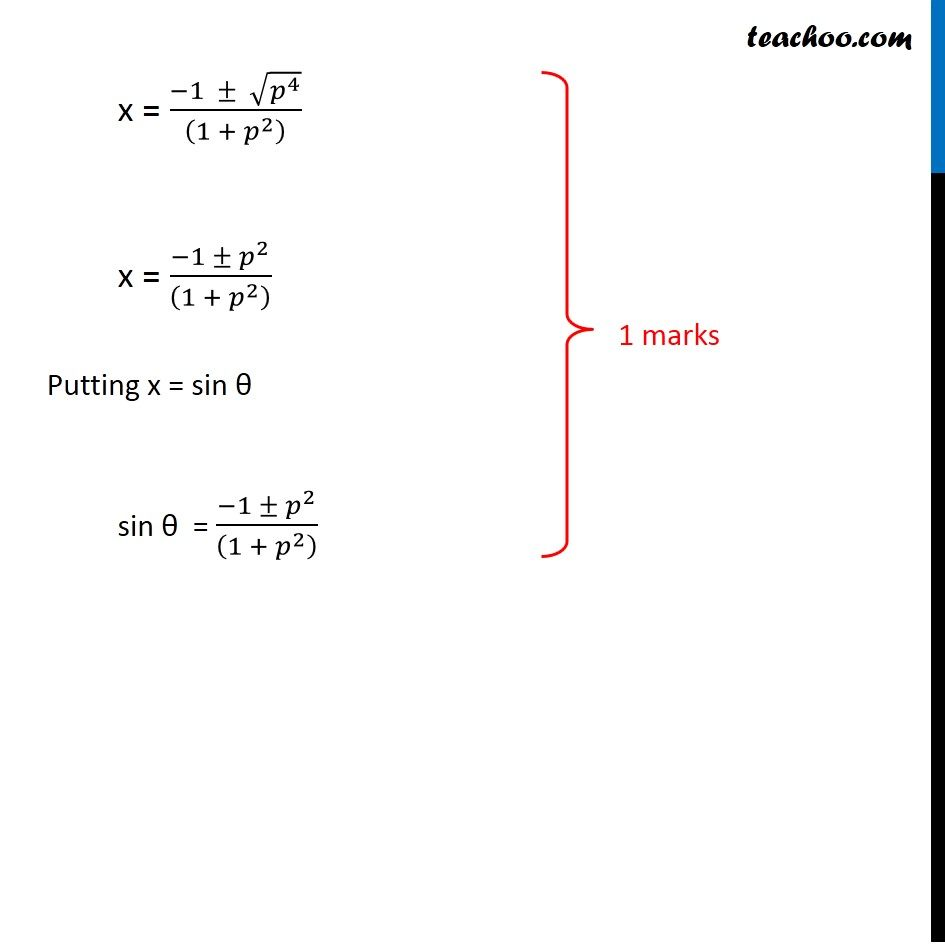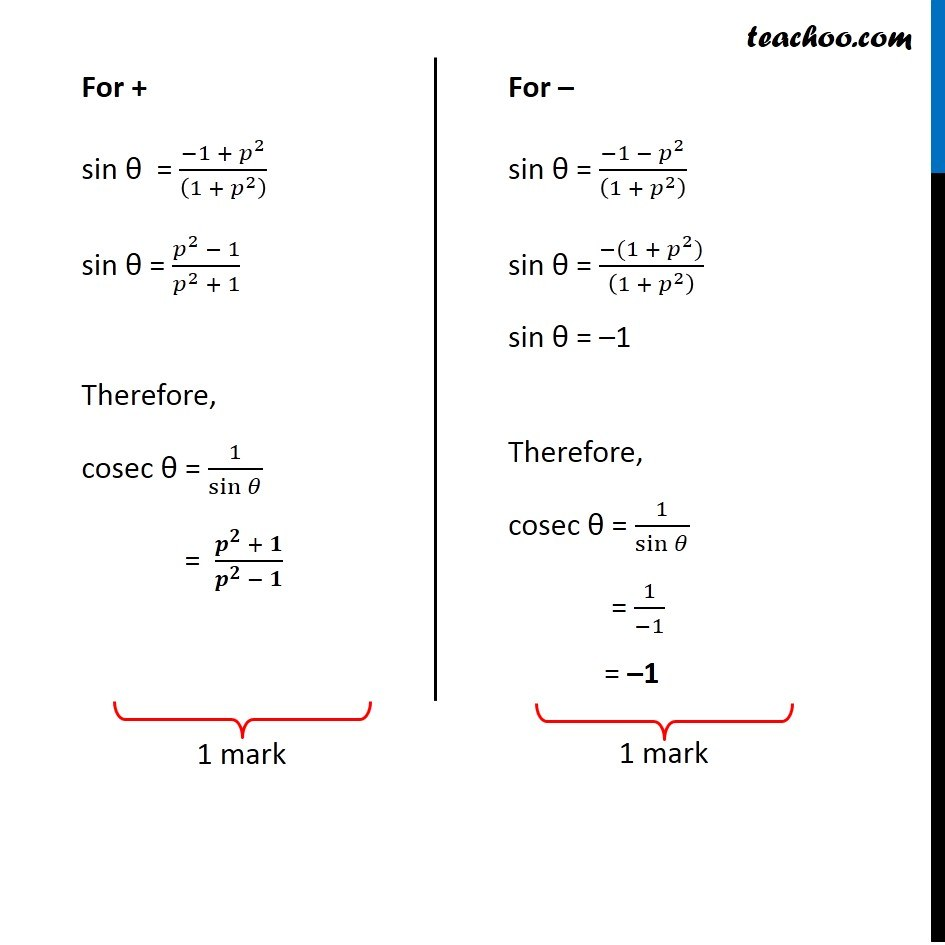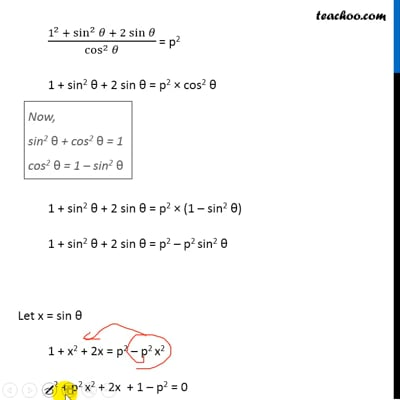This video is only available for Teachoo black users

### Transcript

Question 30 If sec θ + tan θ = p, then find the value of cosec θ Given sec θ + tan θ = p Converting sec θ and tan θ in sin θ and cos θ 1/cos⁡𝜃 + sin⁡𝜃/cos⁡𝜃 = p (1 + sin⁡𝜃)/cos⁡𝜃 = p We need to find cosec θ, To find cosec θ , we convert everything into sin θ So, we square both sides ((1 + sin⁡𝜃)/cos⁡𝜃 )^2 = p2 (1^2 + sin^2⁡𝜃 + 2 sin⁡𝜃)/cos^2⁡𝜃 = p2 1 + sin2 θ + 2 sin θ = p2 × cos2 θ Now, sin2 θ + cos2 θ = 1 cos2 θ = 1 – sin2 θ 1 + sin2 θ + 2 sin θ = p2 × (1 – sin2 θ) 1 + sin2 θ + 2 sin θ = p2 – p2 sin2 θ Let x = sin θ 1 + x2 + 2x = p2 – p2 x2 x2 + p2 x2 + 2x + 1 – p2 = 0 (1 + p2) x2 + 2x + (1 – p2) = 0 Comparing with ax2 + bx + c a = (1 + p2) , b = 2, c = (1 – p2) Now, x = (−𝑏 ± √(𝑏^2 − 4𝑎𝑐))/2𝑎 x = (−2 ± √(2^2 − 4 × (1 + 𝑝^2 ) × (1 − 𝑝^2 ) ))/2(1 + 𝑝^2 ) x = (−2 ± √(4 − 4 × (1 + 𝑝^2 ) × (1 − 𝑝^2 ) ))/2(1 + 𝑝^2 ) x = (−2 ± √4 √(1 − (1 + 𝑝^2 ) × (1 − 𝑝^2 ) ))/2(1 + 𝑝^2 ) x = (−2 ± 2 √(1 − (1 + 𝑝^2 ) × (1 − 𝑝^2 ) ))/2(1 + 𝑝^2 ) x = (−1 ± √(1 − (1 + 𝑝^2 ) × (1 − 𝑝^2 ) ))/((1 + 𝑝^2 ) ) x = (−1 ± √(1 − (1^2 −(𝑝^2 )^2 ) ))/((1 + 𝑝^2 ) ) x = (−1 ± √(1 − (1 − 𝑝^4 ) ))/((1 + 𝑝^2 ) ) x = (−1 ± √(1 − 1 + 𝑝^4 ))/((1 + 𝑝^2 ) ) x = (−1 ± √(𝑝^4 ))/((1 + 𝑝^2 ) ) x = (−1 ± 𝑝^2)/((1 + 𝑝^2 ) ) Putting x = sin θ sin θ = (−1 ± 𝑝^2)/((1 + 𝑝^2 ) ) For + sin θ = (−1 + 𝑝^2)/((1 + 𝑝^2 ) ) sin θ = (𝑝^2 − 1)/(𝑝^2 + 1) Therefore, cosec θ = 1/sin⁡𝜃 = (𝒑^𝟐 + 𝟏)/(𝒑^𝟐 − 𝟏) For – sin θ = (−1 − 𝑝^2)/((1 + 𝑝^2 ) ) sin θ = (−(1 + 𝑝^2))/((1 + 𝑝^2 ) ) sin θ = –1 Therefore, cosec θ = 1/sin⁡𝜃 = 1/(−1) = –1